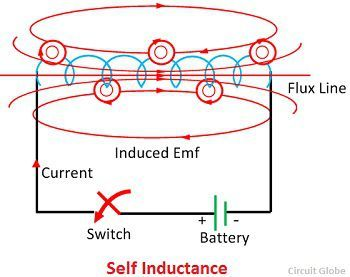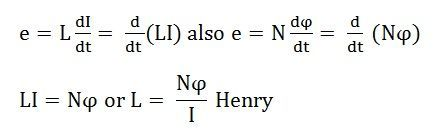# Self Inductance

Definition: Self-inductance or in other words inductance of the coil is defined as the property of the coil due to which it opposes the change of current flowing through it. Inductance is attained by a coil due to the self-induced emf produced in the coil itself by changing the current flowing through it.

If the current in the coil is increasing, the self-induced emf produced in the coil will oppose the rise of current, that means the direction of the induced emf is opposite to the applied voltage.If the current in the coil is decreasing, the emf induced in the coil is in such a direction as to oppose the fall of current; this means that the direction of the self-induced emf is same as that of the applied voltage. Self-inductance does not prevent the change of current, but it delays the change of current flowing through it.

This property of the coil only opposes the changing current (alternating current) and does not affect the steady current that is (direct current) when flows through it. The unit of inductance is Henry (H).

Expression For Self Inductance

You can determine the self-inductance of a coil by the following expressionThe above expression is used when the magnitude of self-induced emf (e) in the coil and the rate of change of current (dI/dt) is known.

Putting the following values in the above equations as e = 1 V, and dI/dt = 1 A/s then the value of Inductance will be L = 1 H.

Hence, from the above derivation, a statement can be given that a coil is said to have an inductance of 1 Henry if an emf of 1 volt is induced in it when the current flowing through it changes at the rate of 1 Ampere/second.

The expression for Self Inductance can also be given as:where,
N – number of turns in the coil
Φ – magnetic flux
I – current flowing through the coil

From the above discussion, the following points can be drawn about Self Inductance

• The value of the inductance will be high if the magnetic flux is stronger for the given value of current.
• The value of the Inductance also depends upon the material of the core and the number of turns in the coil or solenoid.
• The higher will be the value of the inductance in Henry, the rate of change of current will be lower.
• 1 Henry is also equal to 1 Weber/ampere

The solenoid has large self-inductance.

### 8 thoughts on “Self Inductance”

1. In the case where it is DC and the winding count changes increasing over time the current will in fact be lowered as the Magnetic flux to ratio has changed causing an EMF to occur that opposes the original current flow. so the comment of a steady state DC is incorrect.

regards,
Donald